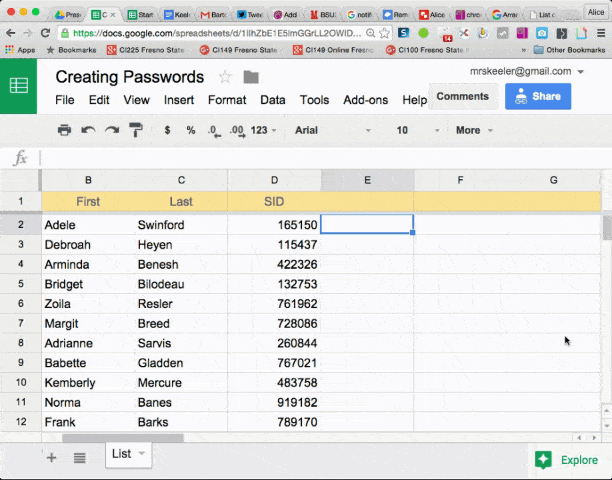# &

The ampersand allows you to concatenate (smash together) values. For example =A2&B2 will smash together the contents of cell A2 with B2.

# LEFT

=LEFT(cell, number of characters) will return the set number of characters from the left side of the text string. For example if A2 has a value of Rhonda, then =LEFT(A2,3) will return “Rho.”

# RIGHT

=RIGHT(cell, number of characters) will return the set number of characters from the right side of the text string. For example if A2 has a value of Rhonda then =RIGHT(A2, 3) will return “nda.”

# MID

=MID(cell, starting character position, number of characters) will return a set of characters from the middle of the text string. For example if A2 has a value of Rhonda then =MID(A2, 2,3) will return “hon.” Three characters starting at the 2nd character.

# RANDBETWEEN

=RANDBETWEEN(min value, max value) will generate a random number between two values. For example =RANDBETWEEN(111111,999999) will create a random 6 digit number. Once you’ve generated the number make sure you highlight the column, copy, right click and choose paste special values. This will remove the formula and replace it with the text value. Otherwise the random number will continue to generate on every edit.

# LOWER

=LOWER(cell) will change all of the characters to lower case. =UPPER(cell) will change all of the characters to upper case.

# For Example

Here is the recent request:

“First initial from first name column, last name, last four digits from a seven digit ID # column”

## Formula

=LEFT(A2,1)&B2&RIGHT(C2,4)

Notice the use of concatenate (&) to join together the three desired values.

# Another Example

I helped someone else generate passwords for younger children. For those passwords we wanted to take a random work and concatenate (smash together) with another random word and then a 2 digit number.

To accomplish this I created a numbered list. In the two columns next to the numbered list, I made list of sight words.# VLOOKUP

=VLOOKUP(cell, lookup range, column) will look up a value from a table and return a corresponding value. Use absolute cell referencing on the lookup range. For example of the table of values is Sheet2!A3:D20 then you want to place dollar signs in the range. For example Sheet2!\$A\$3:\$D\$20. This locks down the range so that when you paste the lookup formula, the range does not adjust. The column is not “A” or “B” but rather the column position in the range. For example if the range is Sheet2!\$D\$15:\$J\$99 then 1 refers to column D, 2 refers to column E,…. 7 refers to column J.

Create 2 columns next to student names. One for the first random digit and one for the second random digit. Use the formula =RANDBETWEEN(0,9) in the cells to generate random digits. Click on the column indicators to highlight the columns. Copy the values. Right click, choose paste special, paste values. This will stop randomizing the numbers and freeze the values in the cells.

In the column next to the random numbers you want to look up a random word, concatenate that with another random word, concatenate that with a digit and concatenate that with the other digit.

## Formula

=VLOOKUP(RANDBETWEEN(1,17),words!\$A\$2:\$C\$18,2)&VLOOKUP(RANDBETWEEN(1,17),words!\$A\$2:\$C\$18,2)&F2&G2

In the above example my list of words are on a tab named “words” and I have a list of 17 values. The F2 and G2 are the random numbers in the columns I made previously. I could have adjusted the formula to not require the columns.
=VLOOKUP(RANDBETWEEN(1,17),words!\$A\$2:\$C\$18,2)&VLOOKUP(RANDBETWEEN(1,17),words!\$A\$2:\$C\$18,2)&RANDBETWEEN(0,9)&RANDBETWEEN(0,9)

Remember to copy the column of passwords, right click to paste special, paste values. This will stop the randomization and provide you with a list of passwords for students.